Brewzor Calculator FREE|不限時間玩工具App

• 419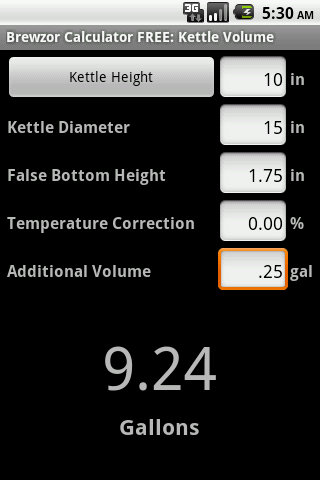Brewzor Calculator is for the home brewer and professional brewer. Hopefully it will help you brew better beer and simplify your brew day.

The following calculators are included:

Strike Temperature: Calculates infusion temperature, volume and water/grain ratio needed for your initial strike.

Mash Infusion: Calculates infusion temperature and volume needed to raise the temperature of the mash.

Decoction Volume: Calculates the decoction volume needed to raise the temperature of the mash.

Batch Sparge: Calculates mash water volumes, water/grain ratio, and grain absorption.

Gravity Correction: Calculates amount of extract or water needed to alter the gravity of a given volume of wort.

Boil Off: Calculates starting, ending, boil off and temperature corrected volumes for a boil.

Hop Bitterness: Calculates estimated IBUs for a given wort and hop schedule.

Hop Alpha Correction: Calculates the new hop weight needed to provide the same IBUs of the original hop when the new hop is a different alpha and or hop form (Pellet, Plug, or Leaf).

Kettle Volume: Calculates volumes in a cylindrical vessel.

Yeast Pitching Rate: Calculates the number of cells needed for optimal fermentation. Allows for multiple steps when additional cells must be grown.

Carbonation: Calculates CO2 pressure, and priming sugar weights needed to carbonate beer.

Gyle: Calculates the amount of gyle needed to carbonate beer.

Efficiency: Calculates brewhouse efficiency.

Hydrometer Correction: Calculates the corrected gravity when a hydrometer sample is not at the calibration temperature.

Alcohol / Attenuation: Calculates real and apparent attenuation as well as alcohol by weight and volume.

Refractometer: Calculates corrected gravity values when taking readings with a refractometer.

Styles: BJCP Style Guide

Color Estimate: Calculates estimated color for a given SRM or EBC.

Unit Conversions: Common unit conversions.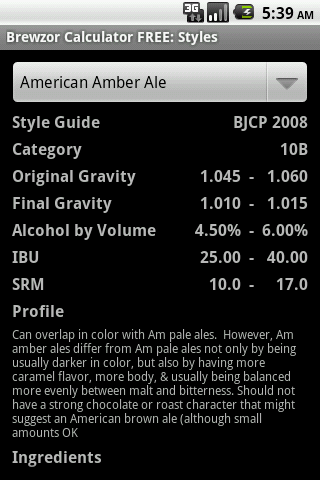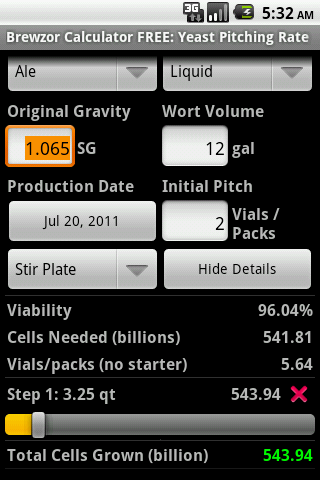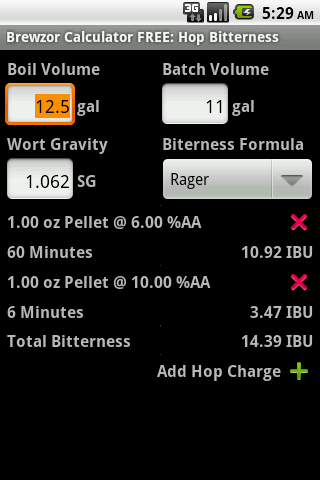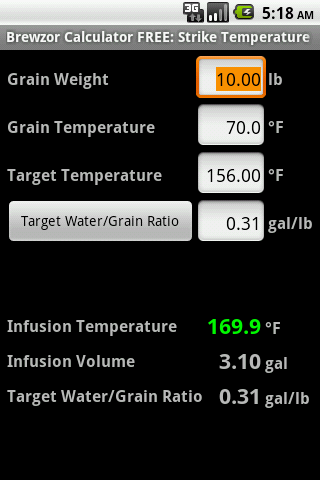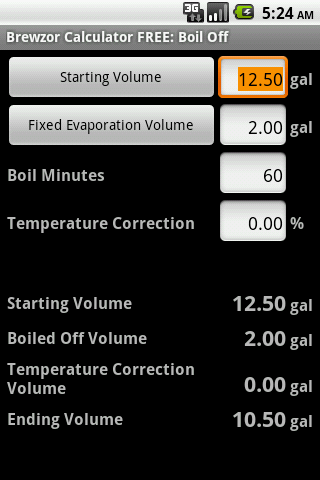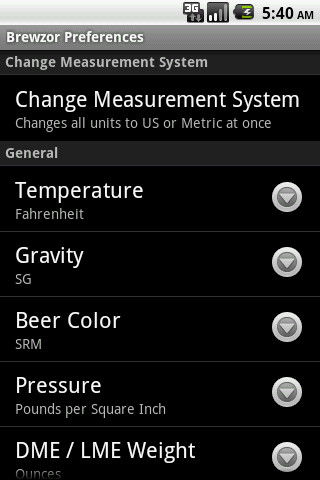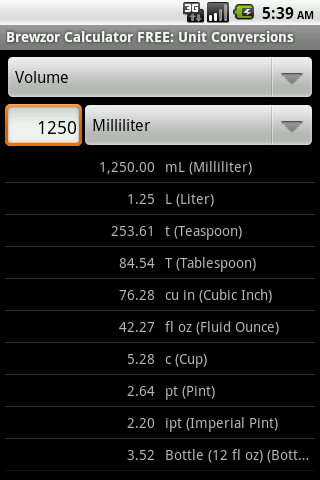Brewzor Calculator FREE 線上APP手遊玩免費

不限時間玩Brewzor Calculator FREE App免費

App國家分佈App支援系統App上架版本費用評價App上架時間更新時間